• MATLAB编写的直方图均衡化、邻域平均与中值滤波、同态增晰和三维图像复原。文件包含matlab源代码和相关的课程作业word文档。
• 车牌识别 数字图像报告 有完整代码 但识别成功率不是特别高
• 西安电子科技大学《 数字图像处理大作业全部源代码；电子工程学院；通信工程学院；人工智能学院；空间科学与技术学院等
• # 数字图像处理——基于GUI设计 对图像进行打开，保存，退出系统，并且能实现放大，缩小，裁剪，旋转，反色，锐化，初始化，添加滤波、噪声、图像的转换包括原图到二值、原图到灰度、灰度到二值，图像的直方图显示...
• 数字图像处理大作业 内容 Canny 边缘检测 OTSU 图像分割方法 OpenCV + Python 实现人脸检测
• 开始\图像类型变换\图形几何运算\图像变换\图像添加噪声\图像复原\图像增强\图像分割
• 北航宇航学院数字图像处理大作业二，VC6.0平台编写
• 一些比较基础的matlab图像处理，包含直方图，图像复原，图像增强等，实在不知道怎么写了，反正你懂的，就是期末那点事，拿去给有需要的同学参考参考吧
• 代数运算的特点及应用 * 5.4.1 一个简单的图像叠加隐写 5.4.2 图像三维重建 5.4 图像的简单应用例子 * 5.4.1 一个简单的图像叠加隐写 图像隐藏是指媒体信息的相互隐藏常见的有数字水印和图像的信息伪装等 ...
• 数字处理大作业，用于处理图像，帮助学生理解各种图像处理之后的效果，指导学习用
• 中科院期末王伟强数字图像处理大作页，用MATLAB实现了冈萨雷斯书中的部分程序，带有可视化的界面，可以调节参数，实现的功能简要概括如下：灰度变换与图像锐化，滤波（低通，高通，中值，维纳滤波），噪声模型，...
• USTC数字图像处理大作业，SA11级同学亲情奉献
• 2018年国科秋季学期数字图像处理大作业——使用数字图像处理原理设计算法，配置使用QT设计UI界面设计Photoshop软件。
• BIT数字图像处理大作业——纯C++实现车道线检测本文禁止转载，违者必究！1. 前言：2. 基本思路：3. 灰度图变换：4. 直方图均衡化：5. 阈值分割：6. 中值滤波：7. 边缘检测：8. 直线检测：9. 后续思路：获取完整项目...


BIT数字图像处理大作业——纯C++实现车道线检测
本文禁止转载，违者必究！1. 前言：2. 基本思路：3. 灰度图变换：4. 直方图均衡化：5. 阈值分割：6. 中值滤波：7. 边缘检测：8. 直线检测：9. 后续思路：获取完整项目代码：

本文禁止转载，违者必究！
1. 前言：
没错这又是大作业，这次是数字图像处理的作业：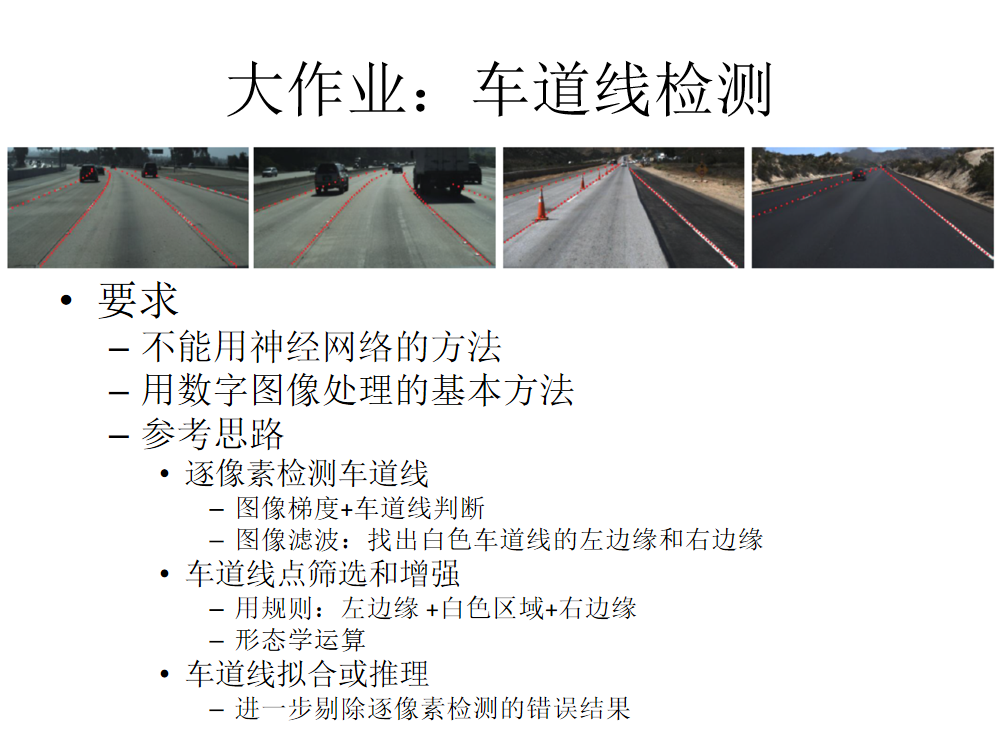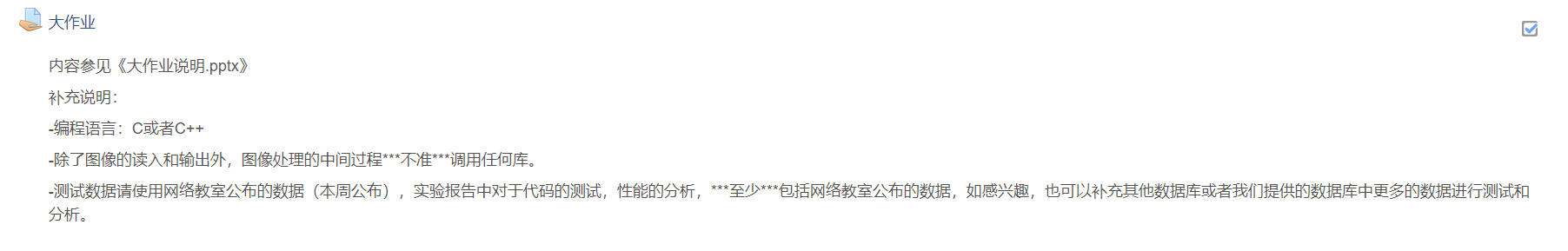本来打算用 LaneNet 搞定，结果发现要求是：
不能用神经网络（即不能用图像分割算法了）；除读取和显示图像，不能用Opencv（即不能调包）；
所以只能老老实实手写那些图像处理代码了。
2. 基本思路：
目前的基本思路如下：
将图像转到灰度图；使用直方图均衡化预处理图像；使用阈值分割方法绘制车道线二值图；对二值图使用中值滤波去除噪点；对二值图使用边缘检测算子进行滤波操作；使用霍夫变换进行直线检测；使用聚类算法聚集直线束（还没写）；
原图：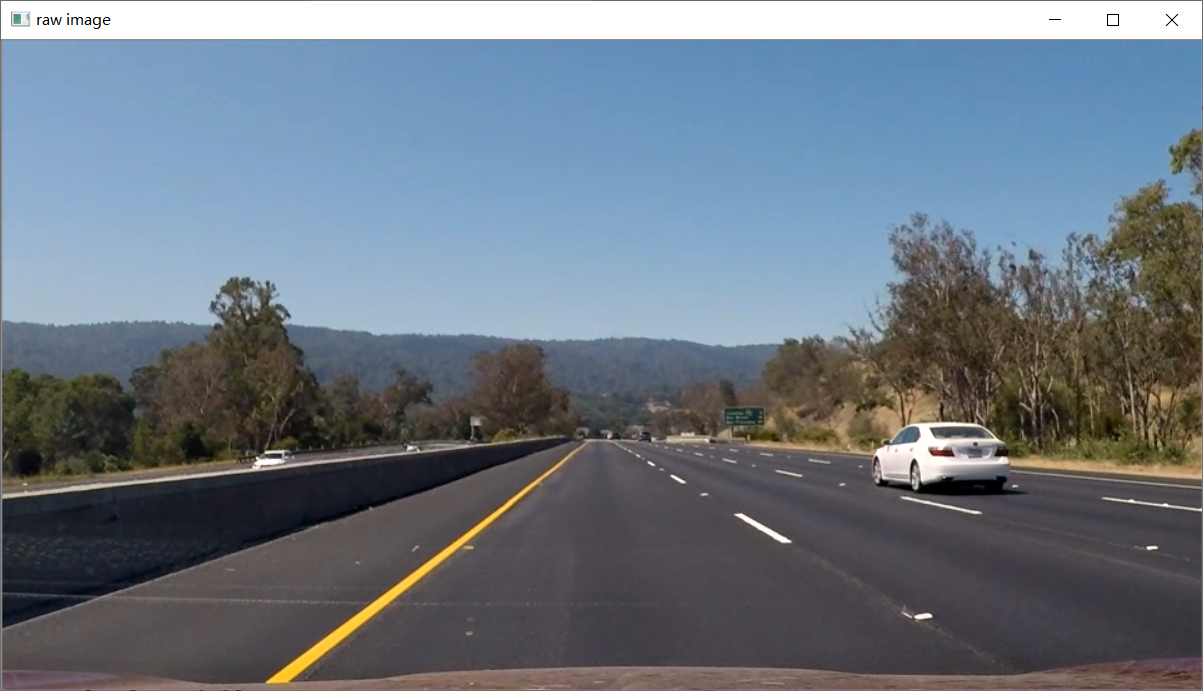3. 灰度图变换：
cv::Mat Color2Gray(cv::Mat src_image)
{
cv::Mat gray_image(src_image.rows, src_image.cols, CV_8UC1);
if (src_image.channels() != 1)
{
for (int i = 0; i < src_image.rows; i++)
for (int j = 0; j < src_image.cols; j++)
gray_image.at<uchar>(i, j) = (src_image.at<cv::Vec3b>(i, j) + src_image.at<cv::Vec3b>(i, j) + src_image.at<cv::Vec3b>(i, j)) / 3;
}
else
gray_image = src_image.clone();
return gray_image;

效果：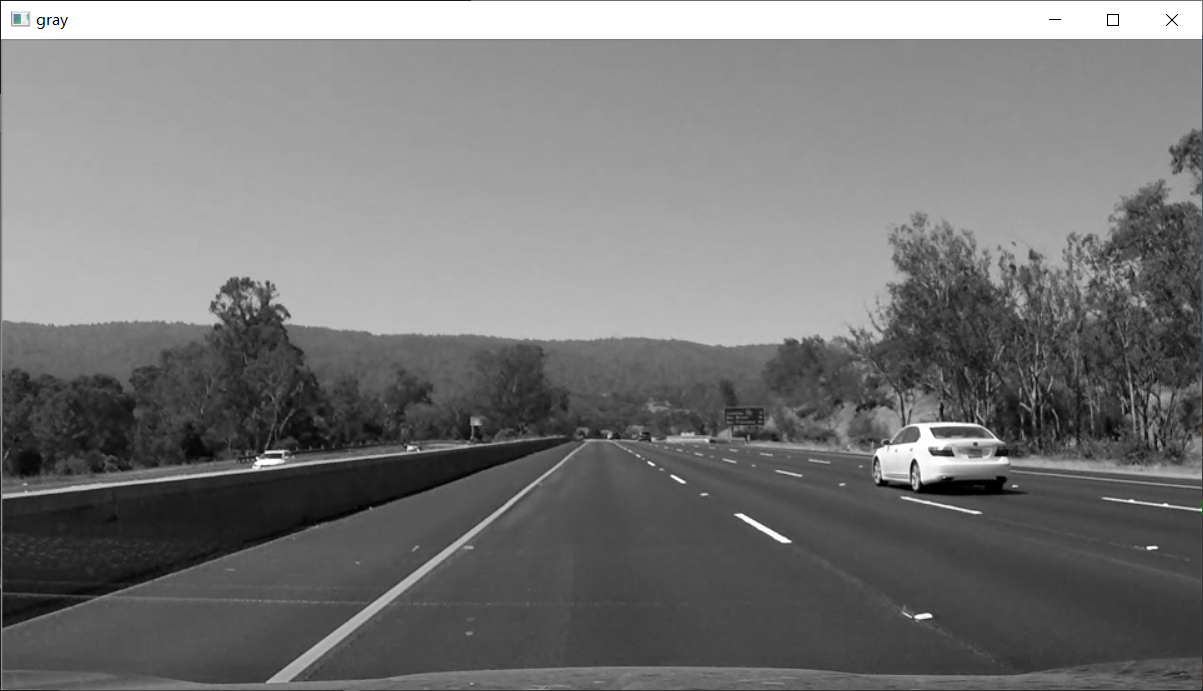4. 直方图均衡化：
cv::Mat equalize_hist(cv::Mat input) {
cv::Mat output = input.clone();
int gray_sum = input.cols * input.rows;
int gray = { 0 };  //记录每个灰度级别下的像素个数
double gray_prob = { 0 };  //记录灰度分布密度
double gray_distribution = { 0 };  //记录累计密度
int gray_equal = { 0 };  //均衡化后的灰度值
//统计每个灰度下的像素个数
for (int i = 0; i < input.rows; i++)
{
uchar* p = input.ptr<uchar>(i);
for (int j = 0; j < input.cols; j++)
{
int vaule = p[j];
gray[vaule]++;
}
}
//统计灰度频率
for (int i = 0; i < 256; i++)
{
gray_prob[i] = ((double)gray[i] / gray_sum);
}
//计算累计密度
gray_distribution = gray_prob;
for (int i = 1; i < 256; i++)
{
gray_distribution[i] = gray_distribution[i - 1] + gray_prob[i];
}

//重新计算均衡化后的灰度值
for (int i = 0; i < 256; i++)
{
gray_equal[i] = (uchar)(255 * gray_distribution[i] + 0.5);
}
//直方图均衡化,更新原图每个点的像素值
for (int i = 0; i < output.rows; i++)
{
uchar* p = output.ptr<uchar>(i);
for (int j = 0; j < output.cols; j++)
{
p[j] = gray_equal[p[j]];
}
}
return output;
}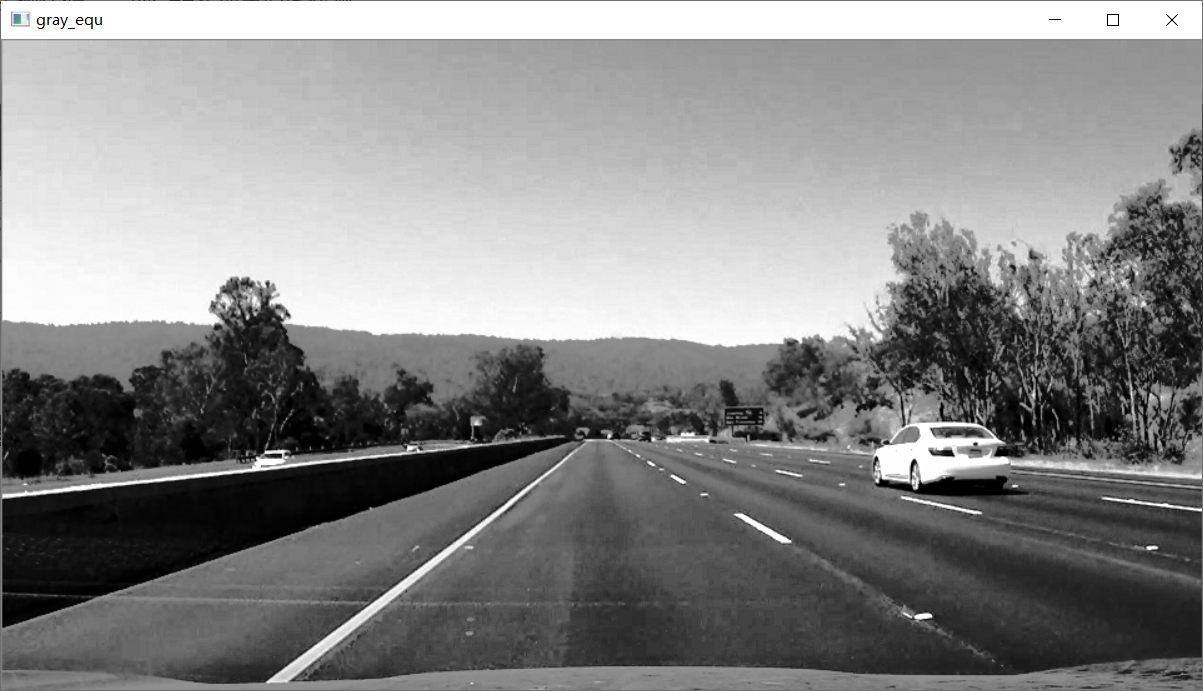5. 阈值分割：
cv::Mat Image2Binary(cv::Mat src_image, int threshold, float init_h, float end_h) {
int value;
float start_i = init_h*float(src_image.rows);
float end_i = end_h * float(src_image.rows);
cv::Mat binary_image(src_image.rows, src_image.cols, CV_8UC1);
for (int i = 0; i < src_image.rows; i++) {
for (int j = 0; j < src_image.cols; j++) {
value = src_image.at<uchar>(i, j);
if (value > threshold && i > start_i && i < end_i) {
binary_image.at<uchar>(i, j) = 255;
}
else {
binary_image.at<uchar>(i, j) = 0;
}
}
}
return binary_image;
}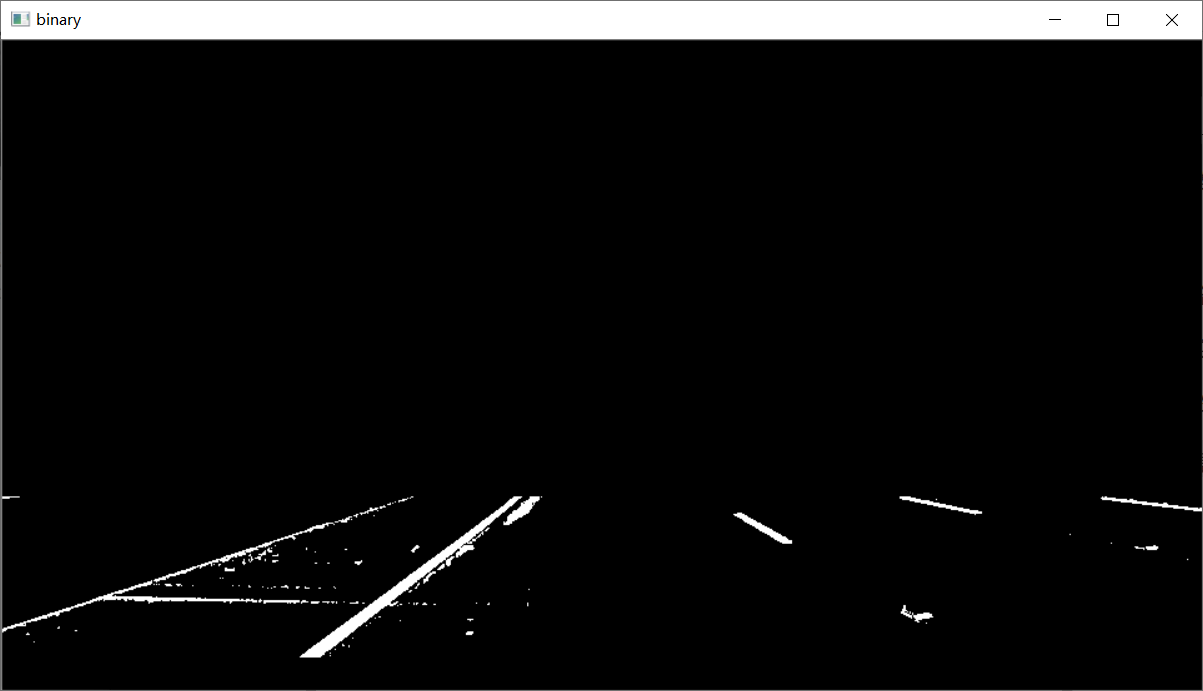6. 中值滤波：
cv::Mat BiMedianBlur(cv::Mat src_image, int size) {
int count_w, count_b, value, ds=(size-1)/2;
cv::Mat result(src_image.rows, src_image.cols, CV_8UC1);
for (int i = 0; i < src_image.rows; i++) {
for (int j = 0; j < src_image.cols; j++) {
count_w = 0;
count_b = 0;
for (int di = -ds; di < ds; di++) {
for (int dj = -ds; dj < ds; dj++) {
if (i + di >= 0 && j + dj >= 0) {
if (i + di < src_image.rows && j + dj < src_image.cols) {
value = src_image.at<uchar>(i+di, j+dj);
if (value == 0) {
count_b += 1;
}
else {
count_w += 1;
}
}
}
}
}
if (count_b > count_w) {
result.at<uchar>(i, j) = 0;
}
else {
result.at<uchar>(i, j) = 255;
}
}
}
return result;
}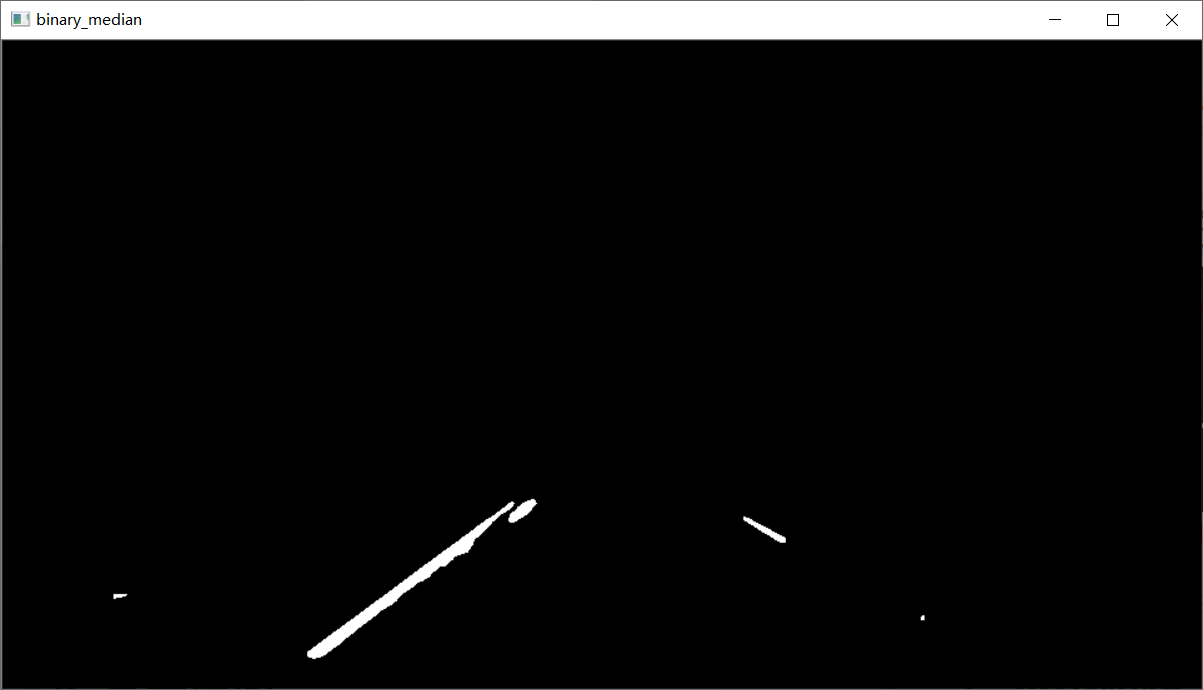7. 边缘检测：
cv::Mat ConvLap(cv::Mat src_image, int thresh) {
int value;
cv::Mat result = cv::Mat::zeros(src_image.size(), CV_8U);
for (int i = 1; i < src_image.rows - 1; i++) {
for (int j = 1; j < src_image.cols - 1; j++) {
value = -4 * src_image.at<uchar>(i, j);
value += src_image.at<uchar>(i - 1, j);
value += src_image.at<uchar>(i + 1, j);
value += src_image.at<uchar>(i, j - 1);
value += src_image.at<uchar>(i, j + 1);
if (value < 0) {
value = -value;
}
if (value > thresh) {
value = 255;
}
else
{
value = 0;
}
result.at<uchar>(i, j) = value;
}
}
return result;
}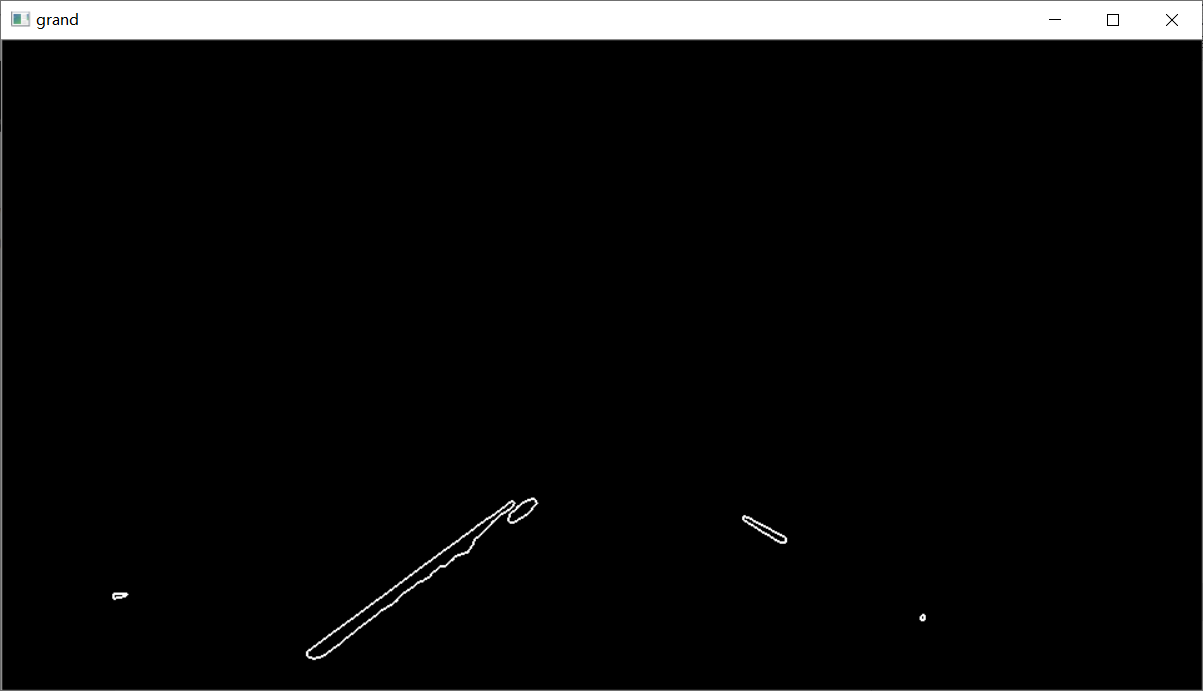8. 直线检测：
std::vector<float> hough_line_v(cv::Mat img, int threshold)
{
int row, col;
int i, k;
//参数空间的参数极角angle(角度)，极径p;
int angle, p;

//累加器
int **socboard;
int *buf;
int w, h;
w = img.cols;
h = img.rows;
int Size;
int offset;
std::vector<float> lines;
//申请累加器空间并初始化
Size = w * w + h * h;
Size = 2 * sqrt(Size) + 100;
offset = Size / 2;
socboard = (int **)malloc(Size * sizeof(int*));
if (!socboard)
{
printf("mem err\n");
return lines;
}

for (i = 0; i < Size; i++)
{
socboard[i] = (int *)malloc(181 * sizeof(int));
if (socboard[i] == NULL)
{
printf("buf err\n");
return lines;
}
memset(socboard[i], 0, 181 * sizeof(int));
}

//遍历图像并投票
int src_data;
p = 0;
for (row = 0; row < img.rows; row++)
{
for (col = 0; col < img.cols; col++)
{
//获取像素点
src_data = img.at<uchar>(row, col);

if (src_data == 255)
{
for (angle = 0; angle < 181; angle++)
{
p = col * cos(angle * PI / 180.0) + row * sin(angle * PI / 180.0) + offset;

//错误处理
if (p < 0)
{
printf("at (%d,%d),angle:%d,p:%d\n", col, row, angle, p);
printf("warrning!");
printf("size:%d\n", Size / 2);
continue;
}
//投票计分
socboard[p][angle]++;

}
}
}
}

//遍历计分板，选出符合阈值条件的直线
int count = 0;
int Max = 0;
int kp, kt, r;
kp = 0;
kt = 0;
for (i = 0; i < Size; i++)//p
{
for (k = 0; k < 181; k++)//angle
{
if (socboard[i][k] > Max)
{
Max = socboard[i][k];
kp = i - offset;
kt = k;
}

if (socboard[i][k] >= threshold)
{
r = i - offset;
//lines_w.push_back(std::);
lines.push_back(-1.0 * float(std::cos(k*PI / 180) / std::sin(k*PI / 180)));
lines.push_back(float(r)/std::sin(k*PI / 180));
count++;
}
}
}
//释放资源
for (int e = 0; e < Size; e++)
{
free(socboard[e]);
}
free(socboard);
return lines;
}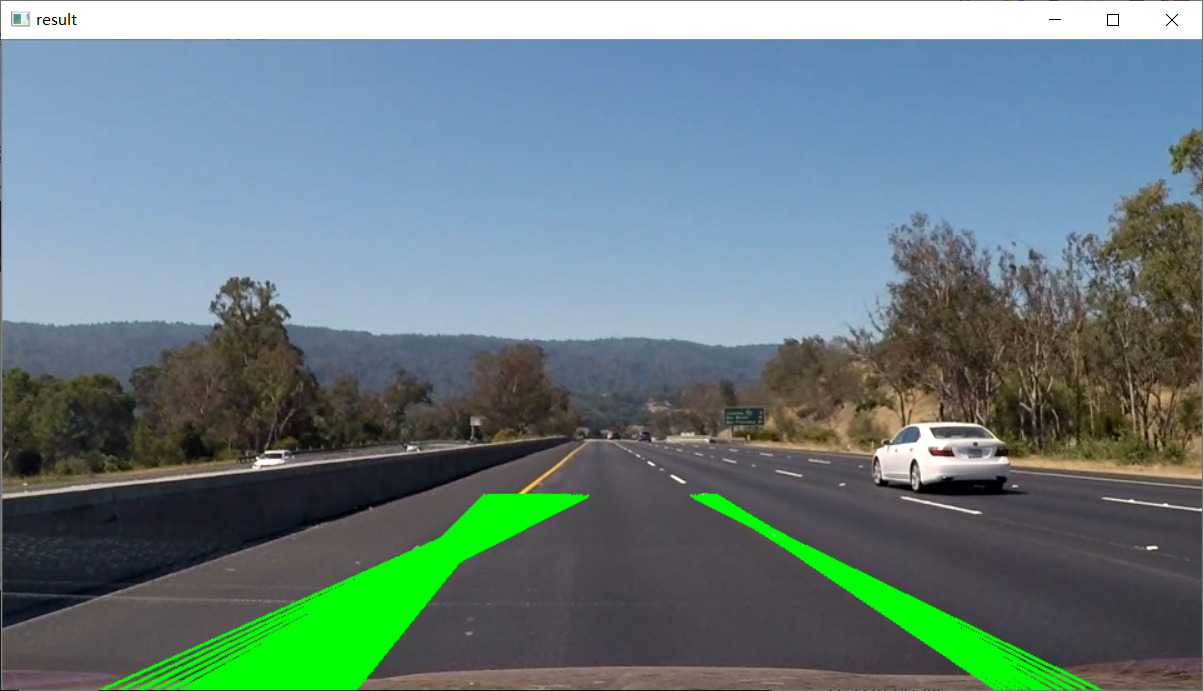9. 后续思路：
可以看到，上面的处理其实效果还ok，但是我们也错过了一些其他的直线。
后续的思路可以观察这张图：我们可以减小中值滤波器大小，二而使用自定义算子去去除水平的干扰线；对不同的连通区域分别生成二值图，然后分别做霍夫变换，防止交叉线的产生；暂时还没有别的思路了。
获取完整项目代码：
感兴趣的同学关注我的公众号——可达鸭的深度学习教程，回复“车道线”获取完整Visual Studio项目：展开全文算法 opencv 计算机视觉 边缘检测 opengl
• 开发环境：matlab R2018b 内容：基于模式匹配的车牌识别 博客：https://blog.csdn.net/qq_44714521/article/details/117255128
• 数字图像处理课程的最后project Matlab的一个窗口集成处理图像
• 图像处理MATLABGUI界面，实现对输入图像的超分辨率重建
•技术
• 北航宇航学院数字图像处理大作业三-遥感图像配准，在VS2013平台上基于opencv2.4.9编写的，SURF和sift算法进行图像配准，并给出旋转角度
• 东南大学数字图像处理期末复习 包括课堂笔记以及试题回忆
• 广东工业大学数字图像处理资源，给需要的师弟和师妹呀
• Digital-Image-Processing-Project 数字图像处理大作业，图像细粒度分类，CUB-200-2011，Peking UniversityPython
• 数字图像处理大作业车牌定位的源代码，测试车牌成功的10张自己拍的图片，还有完整的文档说明。全套的东西直接可以在matlab软件上运行，只需要注意下读取时图片的路径就OK了，全套直接可以上交的作业。超值。车牌定位
• 杭州电子科技大学数字图像处理作业和代码实现，包含源码与结果 珍珠分割
• 完整的实验报告，里面有两道题，最后附有两个实验的代码。
• 大作业 基于模式匹配的车牌识别


大作业 基于模式匹配的车牌识别
1 课程设计任务2 设计框图3 准备工作4 任务流程4.1 图像预处理4.2 图像分割4.2.1 车牌定位(1) 上下定界——水平扫描(2) 左右定界——垂直扫描
4.2.2 车牌字符分割
4.3 图像识别4.3.1 字符细化4.3.2 字符归一化4.3.3 模板匹配
4.4 调试与运行
5 结果5.1 图像预处理结果5.2 图像分割结果5.3 图像识别结果

1 课程设计任务
应用数字图像处理相关知识和技术实现某一应用，如人脸识别、动物识别、水果识别等综合性任务，题目自选。 本文在matlab R2018b开发环境下，实现基于模板匹配的汽车牌照识别。 车牌识别在现代交通管理系统中起着至关重要的作用，具有广阔的应用前景，例如红灯抓拍、超速抓拍、小区记录、公路管理等。如何高效快速地准确识别出汽车牌照成为发展趋势，现如今，随着卷积神经网络的不断改进和壮大，其在车牌识别领域发挥着主要作用。但传统识别作为基础而言，是必须理解并能应用的技术，也是为发展神经网络的奠基石，因此，本文采用传统识别模式匹配技术，进行车牌识别。
2 设计框图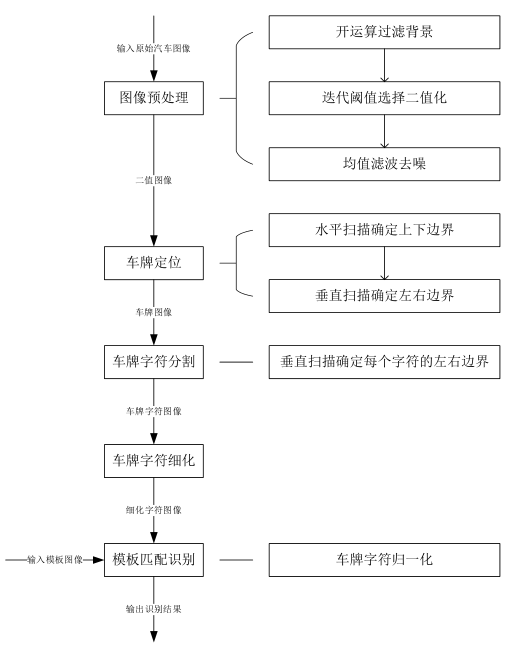3 准备工作
开发环境： windows 10 matlab R2018b制图软件： visio 2013素材示意： 汽车图像素材（来源：百度图片）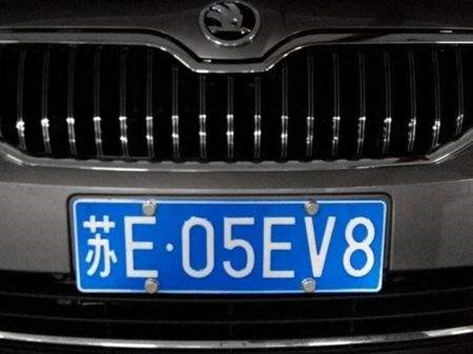模板字符库（来源：CSDN）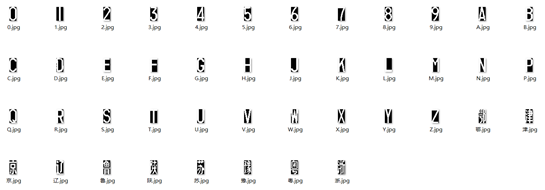模板字符库不含字母“I”、“O”。
4 任务流程
4.1 图像预处理
首先将彩色原始图像image_init读入，利用matlab函数rgb2gray转换成灰度图像image_gray。接着利用matlab函数imopen对image_gray进行背景image_bgd的提取并过滤，得到车牌增强后的图像image_enhance。然后利用自定义函数iterationSeg对image_enhance进行迭代阈值选择，得到二值图像image_bw，此时的二值图像可能含有噪声。最后利用自定义函数average_filter对image_bw进行均值滤波，阈值选择当目标点邻域的8个像素中有3个为白像素时，对目标点予以保留，否则删除。
4.2 图像分割
4.2.1 车牌定位
(1) 上下定界——水平扫描
确定车牌的上边界up和下边界down，，得到分割后的车牌图像image_seg1。
%step5-1 水平扫描确定上下车牌边界
[image_scan1,result1]=projection(image_fill,0);%水平扫描
[width,height]=size(image_fill);%获得图像尺寸
temp1=1; %暂存的上边界
temp2=1; %暂存的下边界
T_max=0; %暂存的车牌宽度
result1_mean=mean(result1);%求水平投影的平均值
result1_min=min(result1);%求水平投影的最小值
result1_level=(result1_mean+result1_min)/2;%求水平投影的平均值
for row=1:width-1
if result1(row)>result1_level
temp2=temp2+1;
else
if(abs(temp2-temp1)>T_max)
up=temp1;
down=temp2;
T_max=abs(temp2-temp1);
end
temp1=row;
temp2=temp1;
end
end

(2) 左右定界——垂直扫描
确定车牌的左边界left和右边界right，得到分割后的车牌图像image_seg2。
%step5-2 垂直扫描确定左右车牌边界
[image_scan2,result2]=projection(image_seg1,1);%垂直扫描
[width,height]=size(image_seg1);%获得图像尺寸
result2_mean=mean(result2);%求垂直投影的平均值
result2_min=min(result2);%求垂直投影的最小值
result2_level=(result2_mean+result2_min)/2;%求垂直投影的平均值
temp1=1;%暂存的左边界
temp2=1;%暂存的第一个字符的右边界
left=temp1;%左边界
T_char=45/140*width;%实际字符宽度
for col=1:height
if result2(col)>result2_level
temp2=temp2+1;
else
if (abs(temp2-temp1)>=T_char&&abs(temp2-temp1)/width<=2*T_char)
left=temp1;
break;
end
temp1=col;
temp2=temp1;
end
end

<1> 方法一：根据垂直扫描确定的车牌左边界，由车牌标准计算右边界。
right=left+ceil((down-up)/2*7+(6*10+12));%右边界

<2> 方法二：垂直扫描分别确定车牌的左右边界。
% temp1=height;%暂存的右边界
% temp2=height;%暂存的最后一个字符的左边界
% right=temp1;
% for col=height:-1:1
%     if result2(col)>result2_level
%         temp2=temp2-1;
%     else
%         if (abs(temp2-temp1)/width>=T_char&&abs(temp2-temp1)/width<=2*T_char)
%             right=temp1;
%             break;
%         end
%             temp1=col;
%             temp2=temp1;
%     end
% end

4.2.2 车牌字符分割
垂直扫描确定字符的左右边界，得到左边界数组temp1和右边界数组temp2。
[image_scan3,result3]=projection(image_seg2,1);%垂直扫描
[width,height]=size(image_seg2);%获得图像尺寸
temp1=ones(1,7); %存放每个字符的左边界
temp2=ones(1,7); %存放每个字符的右边界
for pos=1:7
for col=temp1(pos):height
if result3(col)>T_char/2
temp2(pos)=temp2(pos)+1;
else
if temp1(pos)~=temp2(pos)
temp1(pos+1)=temp2(pos);
temp2(pos+1)=temp2(pos);
break;
end
temp1(pos)=temp1(pos)+1;
temp2(pos)=temp1(pos);
end
end
end
temp2=temp2-1;%多读一列位置，回退

4.3 图像识别
4.3.1 字符细化
根据字符细化的4个条件实现了自定义函数j=thin(i)，即对输入图像i进行细化，并将结果送至j。可以使用系统函数bwmorph(image_seg2,‘thin’,Inf)或thin(image_seg2)对已定位的车牌进行整体细化。再根据字符左边界数组temp1和右边界数组temp2进行字符分割，从而得到每个字符的细化图像〖image_char〗_i （i=1，2，⋯，7）。
4.3.2 字符归一化
实现自定义函数j=normalChar(i)，即对输入图像i进行归一化，并将结果送至j。函数实现包括对输入图像i多余黑色边框的切割，以及利用matlab函数imresize对切割黑边后的i进行缩放至宽45，高90的标准尺寸。对每一个细化后的字符图像进行归一化normalChar(image_char_i)得到图像image_normal_char_i。其中，i=1，2，⋯，7。设置一个90×45×7的矩阵image_normal_char存放归一化后的图像组。
4.3.3 模板匹配
建立模板库字符索引，对待识别字符进行模板匹配。
char_code=char(['0':'9' 'A':'H' 'J':'N' 'P':'Z' '浙豫苏陕鲁辽京']);%部分字符
char_length=36-2+7;
Y=zeros(7,1);
result=zeros(7,1);
for point=1:7
%判断待识别字符所处位置
if point==1
start=36-2+1;
ending=36-2+7;
elseif point==2
start=10+1;
ending=10+26-2;
else
start=1;
ending=36-2;
end
%获取待识别字符图像
imageU=image_normal_char(:,:,point);
[width,height]=size(imageU);
U=sum(sum(imageU==1));
%遍历模板库
for k=start:ending
fname=strcat('D:\1、数字图像处理\2、习题\大作业\字符模板\',char_code(k),'.jpg');
imageT=im2bw(imageT);
imageT=imresize(imageT,[90 45]);
imageV=imageU&imageT;
imageX=xor(imageV,imageU);
imageW=xor(imageV,imageT);
T_char=sum(sum(imageT==1));
V=sum(sum(imageV==1));
X=sum(sum(imageX==1));
W=sum(sum(imageW==1));
TUV=(T_char+U+V)/3;
temp=V/((W/T_char)*(X/U)*sqrt(((T_char-TUV)^2+(U-TUV)^2+(V-TUV)^2)/2));
if temp>Y(point)
Y(point)=temp;
result(point)=k;
end
end
end

4.4 调试与运行
由于字符中，字母“Q”和数字“0”、字母“B”和数字“8”、字母“U”和字母“V”之间十分相似，必须根据它们的字符组成特征，单独进行进一步的区分。定义字符高度小于宽度。可以看出，“Q”和“0”之间的主要区别在于右下角那一块的像素。逐列扫描待识别字符右下角的白像素个数，若细化后的字符仍存在某列的白像素大于3个，即判断这个字符是“Q”，否则为“0”。“B”和“8”的主要区别在于，“B”的最左像素是一条直线，而“8”的最左像素是类似于“ε”的形状，有一定的凹弧。逐列扫描待识别字符的前5列白像素个数，若存在某列白像素个数超过1/4的字符高度，即判断这个字符是“B”，否则为“8”。“U”和“V”的主要区别在于，“U”的左右构造是直线，而“V”的左右构造是斜线。因此逐列扫描待识别字符的前1/2宽度的白像素个数，若存在某列白像素个数超过1/2字符高度，则判断这个字符是“U”，否则为“V”。
5 结果
5.1 图像预处理结果
灰度处理后的图像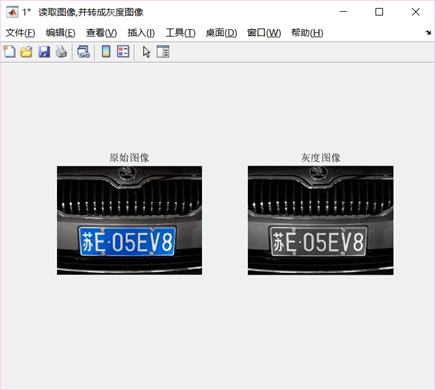过滤背景后的图像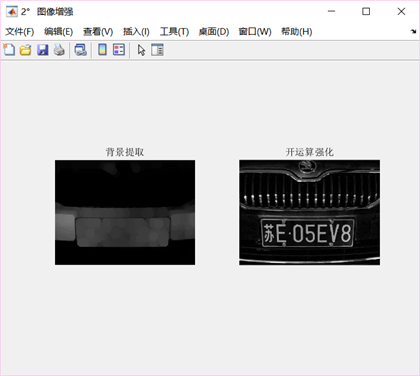二值化——迭代法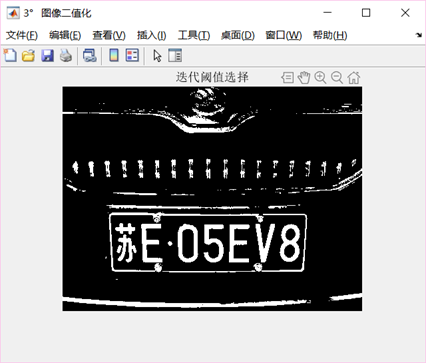去噪——均值滤波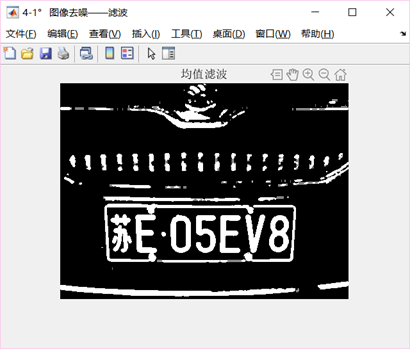5.2 图像分割结果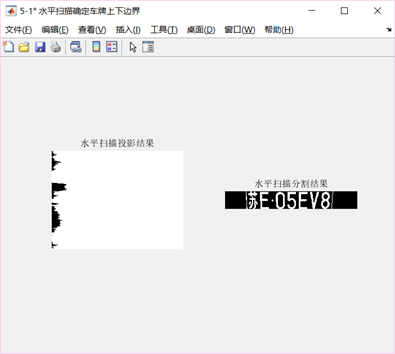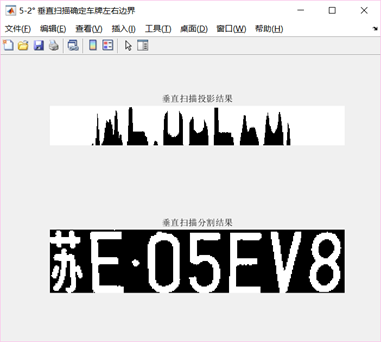车牌字符定界——垂直扫描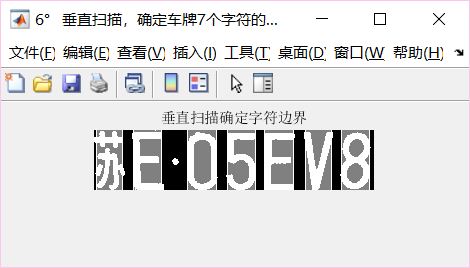5.3 图像识别结果
图像细化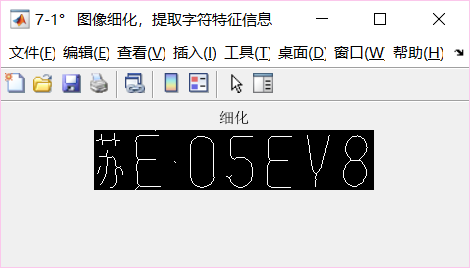分割细化后的车牌字符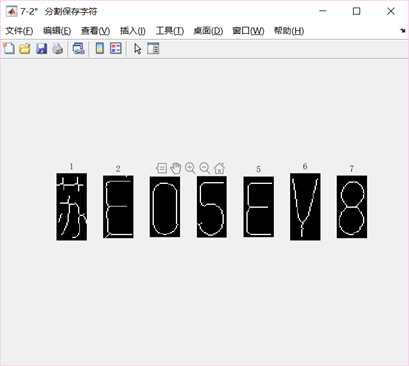字符归一化，同时显示识别结果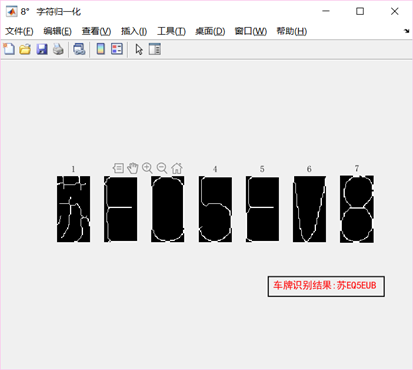进一步调整识别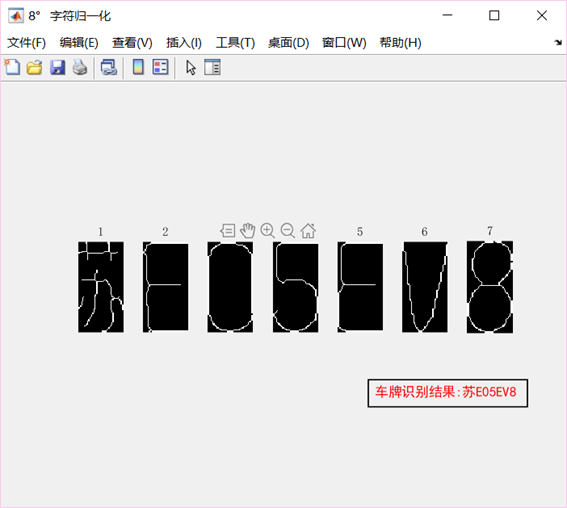展开全文matlab
• 本文件是本人在学习过程中的作业，冈萨雷斯第三版课后习题（6-7）、（6-24）、（6-25）；没有需要勿下载，本人能力有限，如有错误勿喷。...